Next: General case Up: Building the covariance matrix Previous: Offset uncertainty   Contents

### Normalization uncertainty

Let us consider now the case where the calibration constant is the scale factor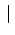, known with a standard uncertainty. Also in this case, for simplicity and without losing generality, let us suppose that the most probable value ofis 1. Then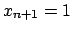, i.e., and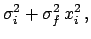. Then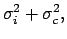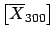(6.35) Cov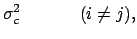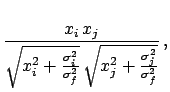(6.36)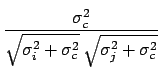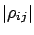(6.37)(6.38)

To verify the results let us consider two independent measurementsand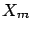; let us calculate the correlated quantitiesand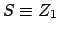, and finally their product (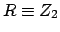) and their ratio (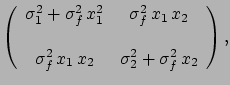):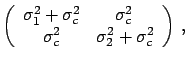(6.39)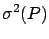(6.40)

It follows that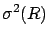(6.41)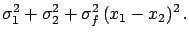(6.42)

Just as an unknown common offset error cancels in differences and is enhanced in sums, an unknown normalization error has a similar effect on the ratio and the product. It is also interesting to calculate the standard uncertainty of a difference in the case of a normalization error: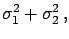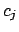(6.43)

The contribution from an unknown normalization error vanishes if the two values are equal.Next: General case Up: Building the covariance matrix Previous: Offset uncertainty   Contents
Giulio D'Agostini 2003-05-15